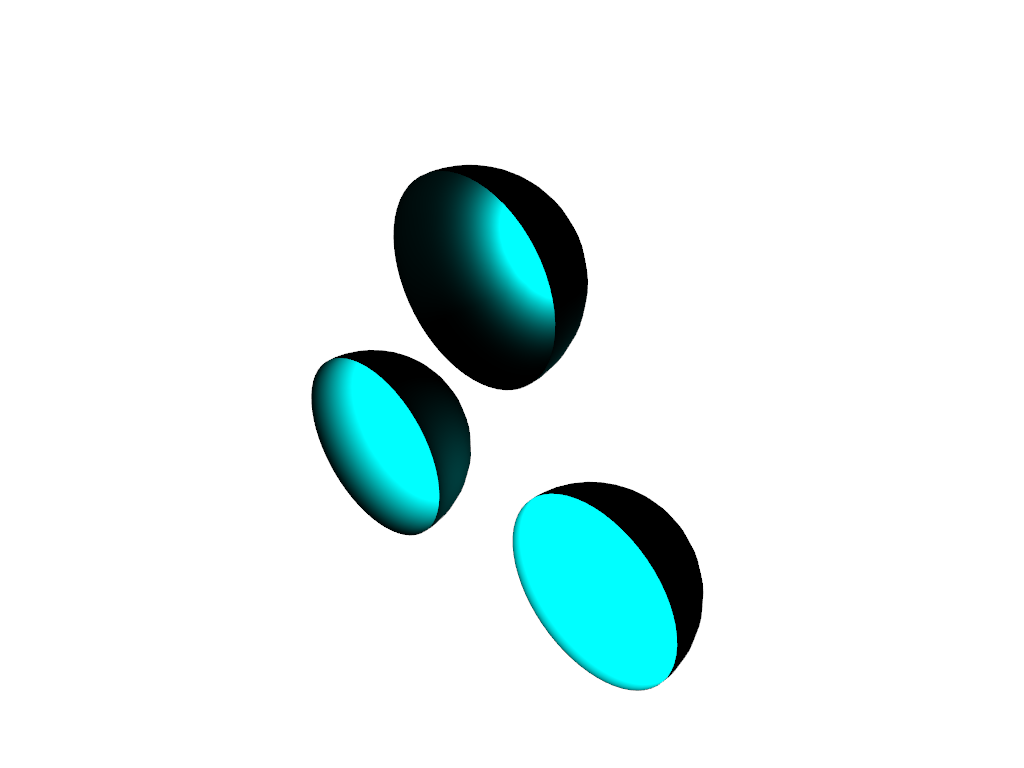# Beam Shape#

The default directional lights are infinitely distant point sources, for which the only geometric customization option is the choice of beam direction defined by the light’s position and focal point. Positional lights, however, have more options for beam customization.

Consider two hemispheres:

```import pyvista as pv

plotter = pv.Plotter()

hemi = pv.Sphere().clip()
hemi.translate((-1, 0, 0), inplace=True)

hemi = hemi.rotate_z(180, inplace=False)

plotter.show()
```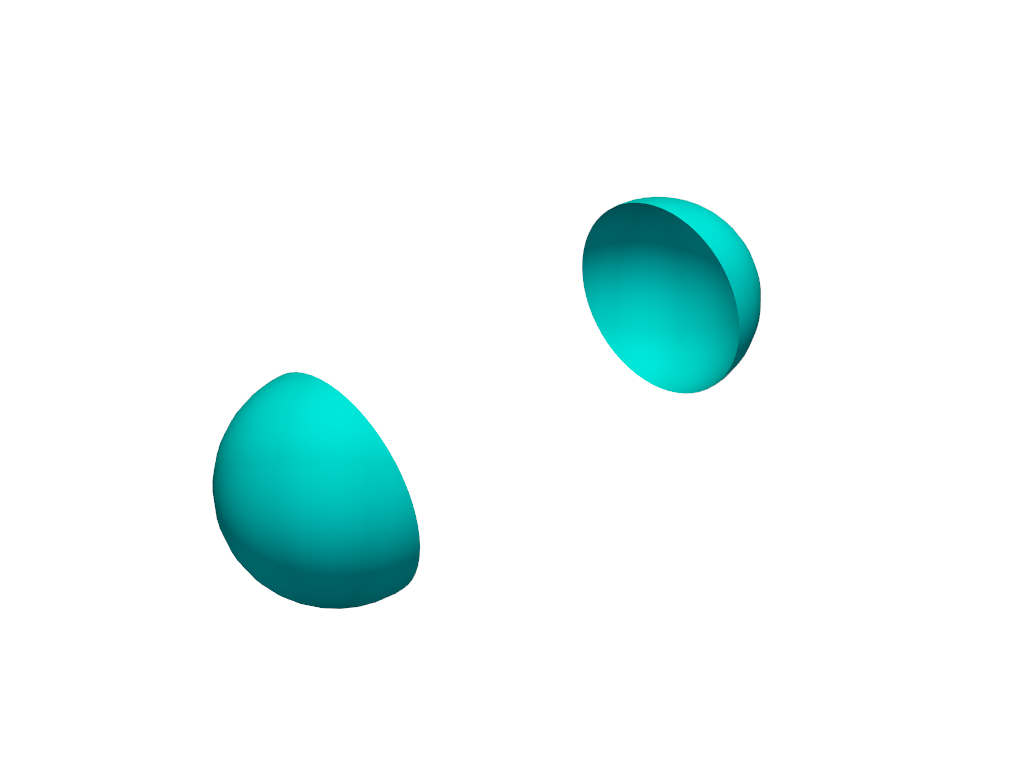We can see that the default lighting does a very good job of articulating the shape of the hemispheres.

Let’s shine a directional light on them, positioned between the hemispheres and oriented along their centers:

```plotter = pv.Plotter(lighting='none')

hemi = pv.Sphere().clip()
hemi.translate((-1, 0, 0), inplace=True)

hemi = hemi.rotate_z(180, inplace=False)

light = pv.Light(position=(0, 0, 0), focal_point=(-1, 0, 0))

plotter.show()
```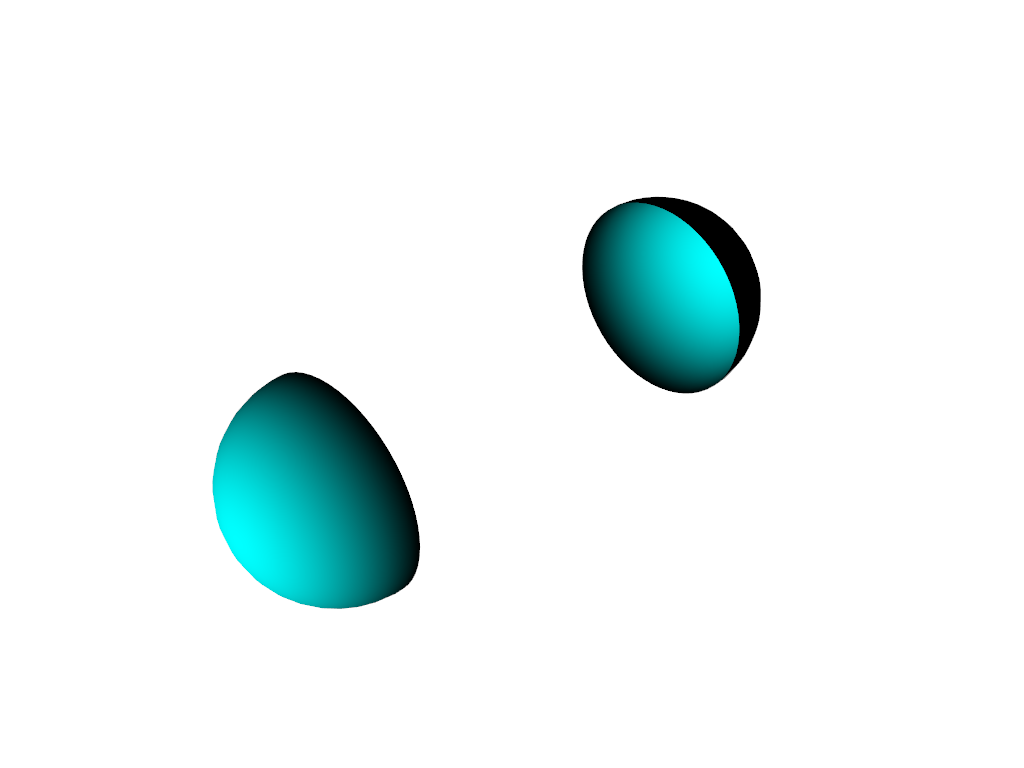Both hemispheres have their surface lit on the side that faces the light. This is consistent with the point source positioned at infinity, directed from the light’s nominal position toward the focal point.

Now let’s change the light to a positional light (but not a spotlight):

```plotter = pv.Plotter(lighting='none')

hemi = pv.Sphere().clip()
hemi.translate((-1, 0, 0), inplace=True)

hemi = hemi.rotate_z(180, inplace=False)

light = pv.Light(position=(0, 0, 0), focal_point=(-1, 0, 0))
light.positional = True
light.cone_angle = 90

plotter.show()
```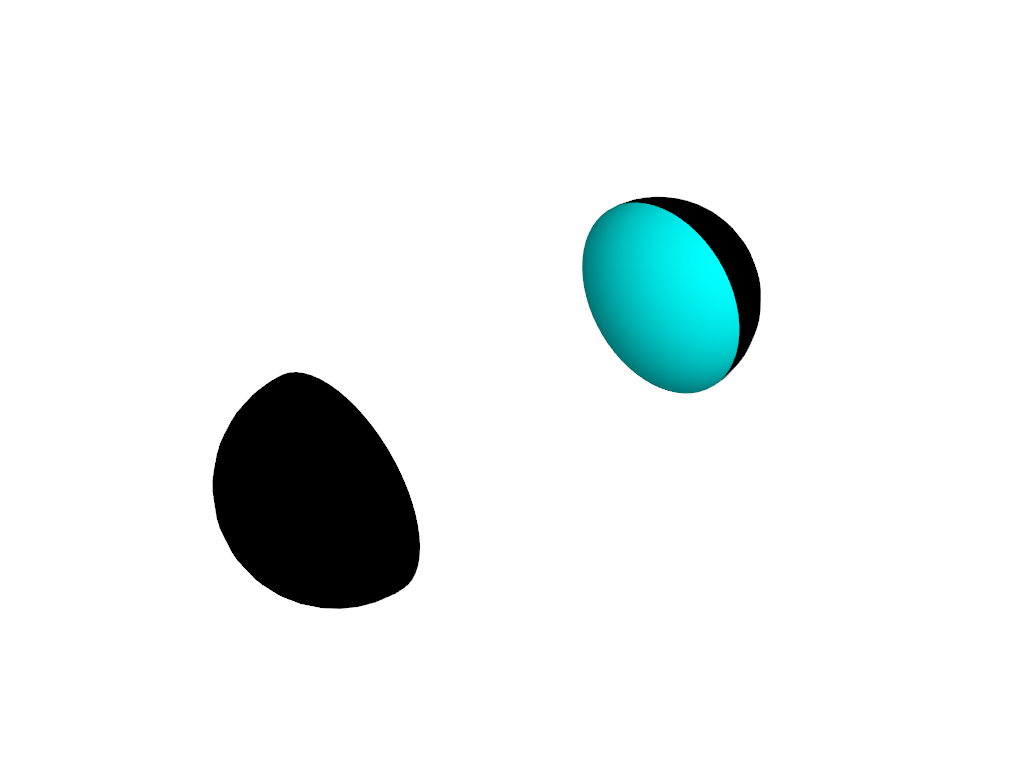Now the inner surface of both hemispheres is lit. A positional light with a cone angle of 90 degrees (or more) acts as a point source located at the light’s nominal position. It could still display attenuation, see the Attenuation example.

Switching to a spotlight (i.e. a positional light with a cone angle less than 90 degrees) will enable beam shaping using the `exponent` property. Let’s put our hemispheres side by side for this, and put a light in the center of each: one spotlight, one merely positional.

```plotter = pv.Plotter(lighting='none')

hemi = pv.Sphere().clip()

offset = 1.5
hemi = hemi.translate((0, offset, 0), inplace=False)

# non-spot positional light in the center of the first hemisphere
light = pv.Light(position=(0, 0, 0), focal_point=(-1, 0, 0))
light.positional = True
light.cone_angle = 90
# add attenuation to reduce cross-talk between the lights
light.attenuation_values = (0, 0, 2)

# spotlight in the center of the second hemisphere
light = pv.Light(position=(0, offset, 0), focal_point=(-1, offset, 0))
light.positional = True
light.cone_angle = 89.9
# add attenuation to reduce cross-talk between the lights
light.attenuation_values = (0, 0, 2)

plotter.show()
```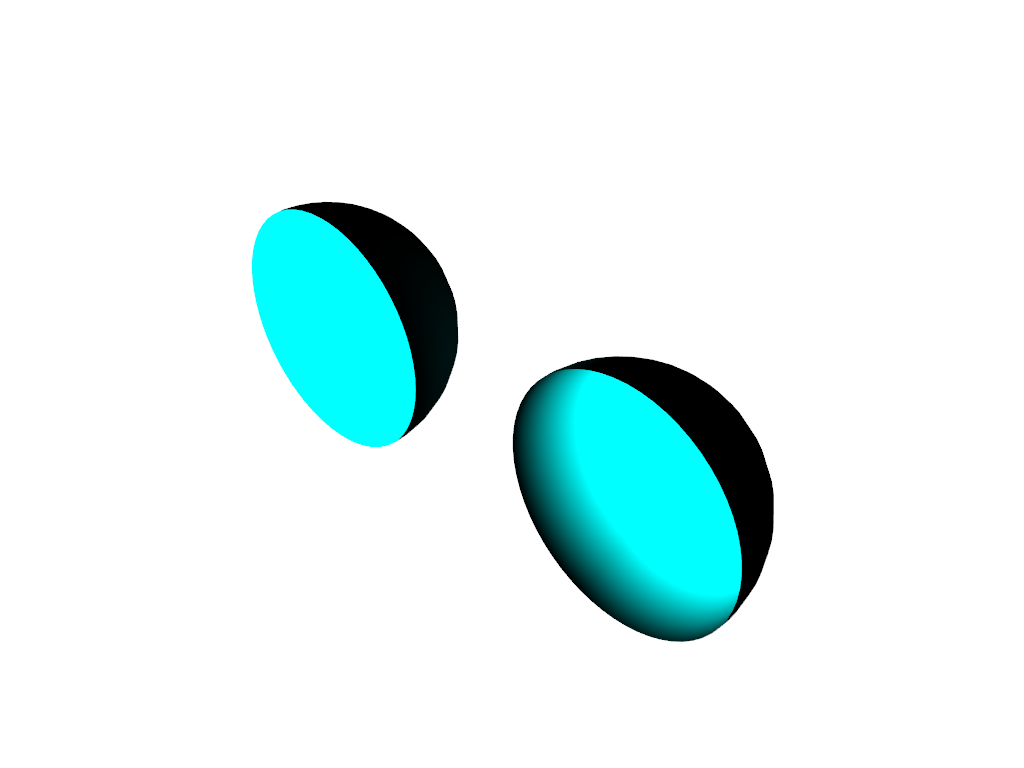Even though the two lights only differ by a fraction of a degree in cone angle, the beam shaping effect enabled for spotlights causes a marked difference in the result.

Once we have a spotlight we can change its `exponent` to make the beam shape sharper or broader. Three spotlights with varying sharpness:

```plotter = pv.Plotter(lighting='none')
hemi_template = pv.Sphere().clip()

centers = [(0, 0, 0), (0, 1.5, 0), (0, 1.5 * 0.5, 1.5 * 3**0.5 / 2)]
exponents = [1, 0.3, 5]

for center, exponent in zip(centers, exponents):
hemi = hemi_template.copy()
hemi.translate(center, inplace=True)

# spotlight in the center of the hemisphere, shining into it
focal_point = center - 1, center, center
light = pv.Light(position=center, focal_point=focal_point)
light.positional = True
light.cone_angle = 89.9
light.exponent = exponent
# add attenuation to reduce cross-talk between the lights
light.attenuation_values = (0, 0, 2)